# Examples#

Examples of several functionalities of the package.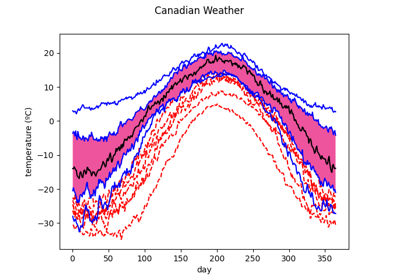Boxplot

Boxplot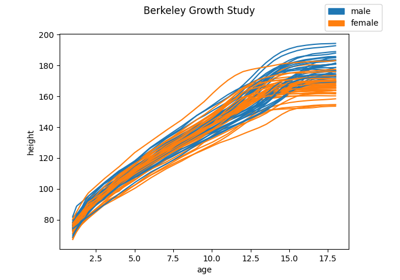Classification methods

Classification methods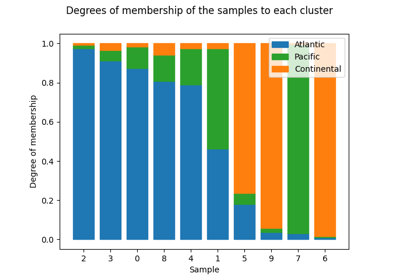Clustering

Clustering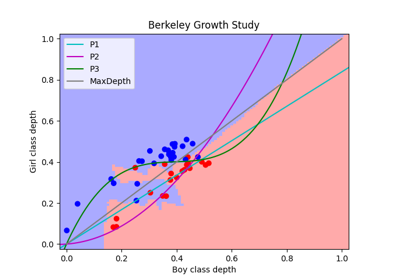Depth based classification

Depth based classification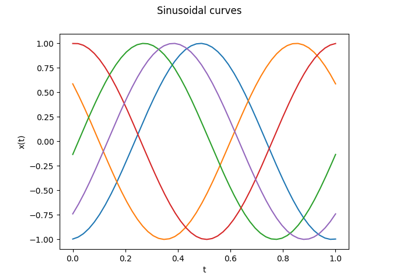Discretized function representation

Discretized function representation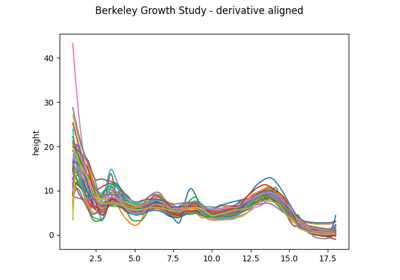Elastic registration

Elastic registration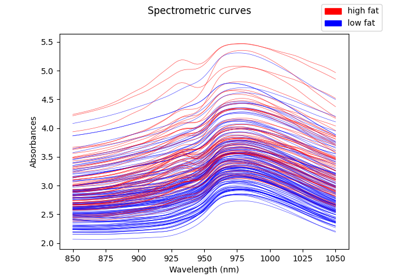Exploring data

Exploring data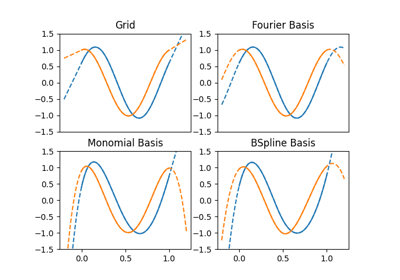Extrapolation

Extrapolation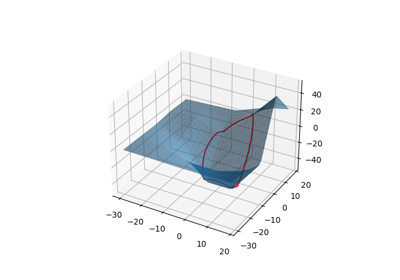Function composition

Function composition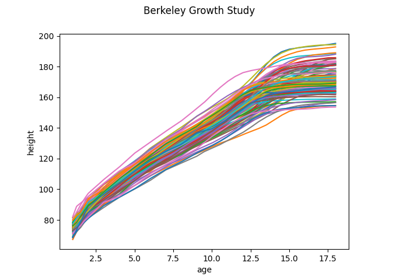Functional Principal Component Analysis

Functional Principal Component Analysis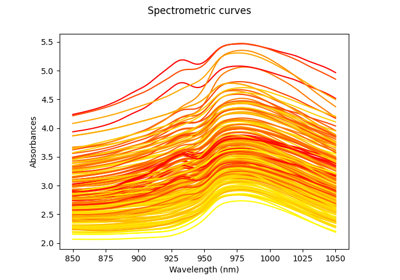Functional Principal Component Analysis Regression.

Functional Principal Component Analysis Regression.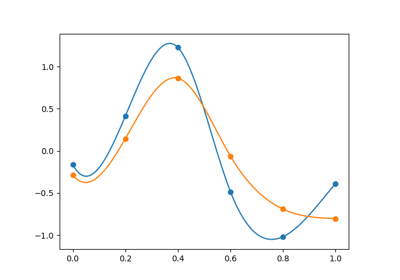Interpolation

Interpolation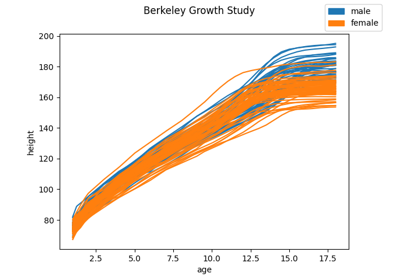K-nearest neighbors classification

K-nearest neighbors classificationKernel Regression

Kernel Regression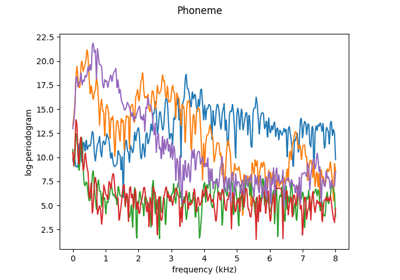Kernel Smoothing

Kernel Smoothing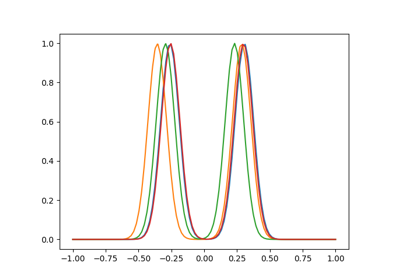Landmark registration

Landmark registration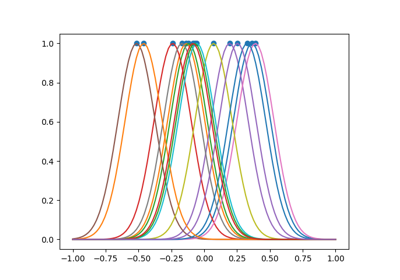Landmark shift

Landmark shift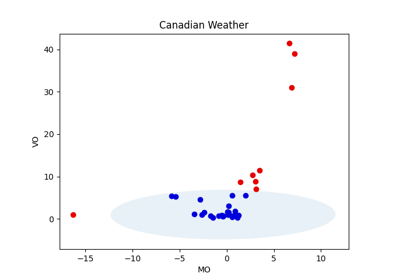Magnitude-Shape Plot

Magnitude-Shape Plot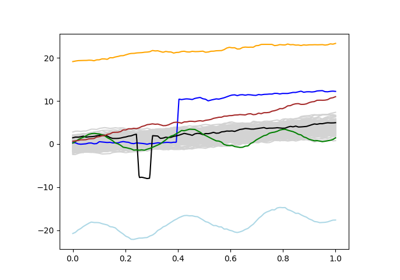Magnitude-Shape Plot synthetic example

Magnitude-Shape Plot synthetic example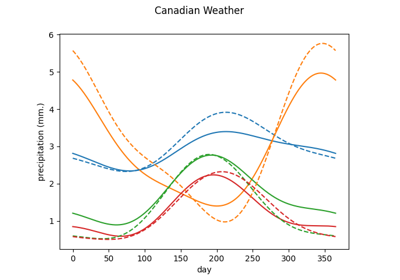Neighbors Functional Regression

Neighbors Functional Regression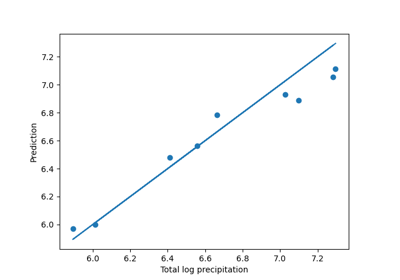Neighbors Scalar Regression

Neighbors Scalar Regression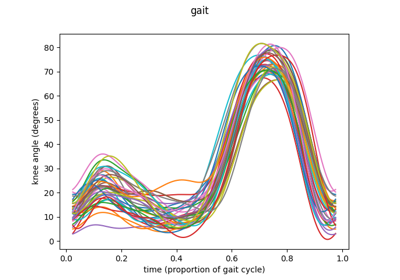One-way functional ANOVA with real data

One-way functional ANOVA with real data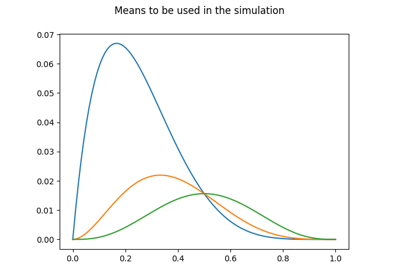One-way functional ANOVA with synthetic data

One-way functional ANOVA with synthetic data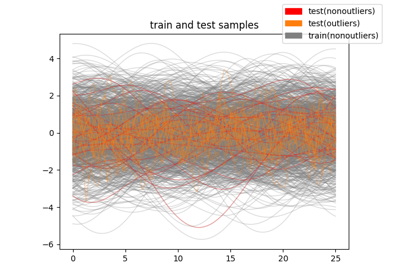Outlier detection with FPCA

Outlier detection with FPCA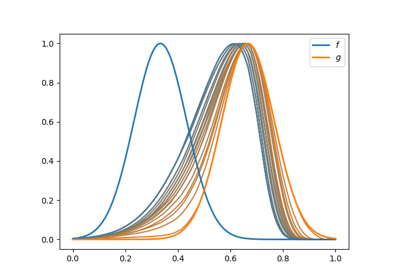Pairwise alignment

Pairwise alignment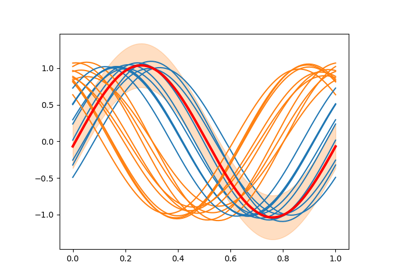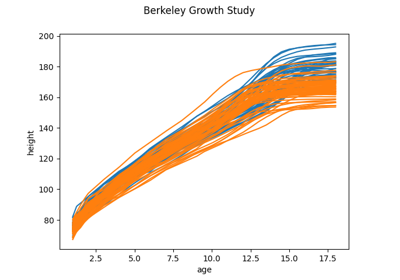Representation of functional data

Representation of functional data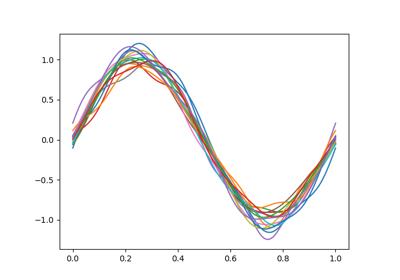Shift Registration

Shift Registration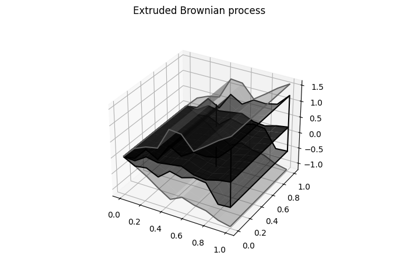Surface Boxplot

Surface Boxplot

# Expand scikit-fda#

Examples of how to expand the functionality of scikit-fda with custom behaviors.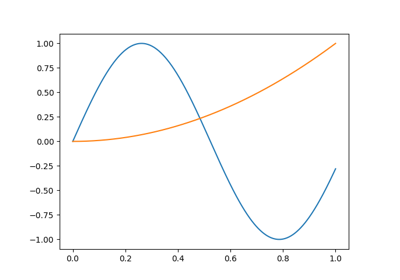Creating a new basis

Creating a new basis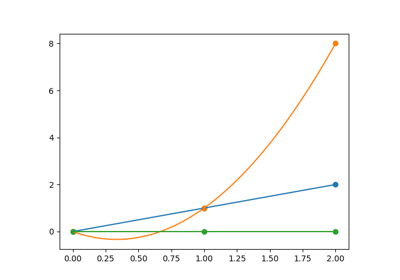Creating a new interpolation or extrapolation strategy

Creating a new interpolation or extrapolation strategy

# ICTAI examples#

Examples of complete analyses showcasing the main functionalities of the scikit-fda package, presented at the 34th IEEE International Conference on Tools with Artificial Intelligence (ICTAI).Meteorological data: data visualization, clustering, and functional PCA

Meteorological data: data visualization, clustering, and functional PCA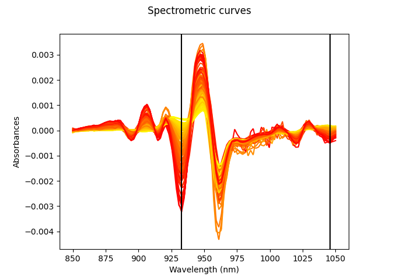Spectrometric data: derivatives, regression, and variable selection

Spectrometric data: derivatives, regression, and variable selection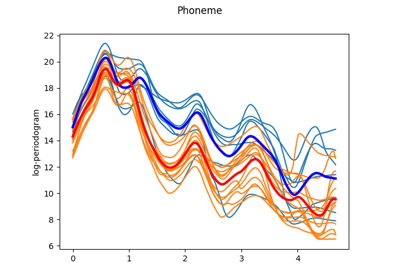Voice signals: smoothing, registration, and classification

Voice signals: smoothing, registration, and classification

Gallery generated by Sphinx-Gallery